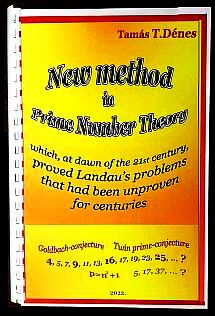Vissza az elõzõ oldalra! Utolsó frissítés: 2022-04-08 3:17
 Tamás T.Dénes New method in Prime Number Theory which, at dawn of the 21st century, proved Landau's problems that had been unproven for centuries   Proving Goldbach's conjecture (especially when even), was for 280 years vainly undertaken. And the even more long-lived twin-prime conjecture is now also theorized via the same venture, ... This volume is thus commended by the Author, who has had the courage to tackle this adventure!Edmund Georg Hermann Landau (1877-1938) was a German mathematician who wrote more than 250 articles on number theory, which had a major impact on the development of the subject. From 1909, he was a professor at the University of Göttingen. At the International Congress of Mathematicians in 1912 (Cambridge, England), Landau listed four problems (conjectures) about prime numbers that had been unproven for centuries, and argued that they were unlikely to be proved in the 20th century by known mathematical methods. His claim has been borne out by life, as these conjectures remained unproven in the 20th century, and are unresolved even today. These four problems have been known as Landau's problems since the 1912 Congress of Mathematicians: 1. Christian Goldbach (1690-1764) - Even Goldbach's conjecture: Each even number which greater than two is the sum of two prime numbers. 2. Twin Primes conjecture: There are infinitely many p primes, where p+2 is also a prime. 3. Adrien-Marie Legendre (1752-1833) - Legendre's conjecture: There is always at least one prime number between two consecutive square numbers. 4. There exists infinitely many prime numbers in the form n2+1. I dedicate the present volume, in which I publish the proofs of these four conjectures, to mark the 110th anniversary of the formulation of Landau's problems, and as a tribute to all the great mathematicians who have made enormous efforts (using traditional number theory) to prove Landau's problems up to the present day. I would like to express my particular respect to Edmund Georg Hermann Landau's insight that "these problems are unlikely to be provable in the 20th century by known mathematical methods"! In his 1993 lecture held in Budapest, Pál Erdõs commented Landau's 1912 problems the following way: "They may be solved in the next century." In the beginning of the 2000s, Professor János Pintz wrote a rather detailed analysis of the results achieved regarding the problems enumerated by Landau, including a 9-page bibliography listing the sources used for the analysis. The proofs presented below are based on my "complementary prime sieve" number theorem published at the dawn of the 21st century, in 2001 (see APPENDIX), and the new methodology based on this theorem ("Dénes type Symmetric Prime Number theorem"). To illustrate the further successful application of this methodology, in the two concluding chapters of the volume, I present - as a kind of mathematical dessert - the proof of the conjecture concerning Mersenne primes, which has not been proved for 400 years, and the proof of the twin primes theorem generalized by myself. All these results, -despite the failure to publish the papers- included in this volume, have deeply confirmed my belief in A. Turing's idea that: "Sometimes it is the people no one can imagine anything of who do the things no one can imagine." (A. M. Turing) C O N T E N T FOREWORD 1. Dénes type Symmetric Prime Number theorem and its application to proof of the Even Goldbach conjecture 2. Proof of the Twin Primes conjecture 3. Proof of the existence of prime number between successive squares 4. Application of the Dénes type Symmetric Prime Number theorem to proof of there exist infinitely many primes of the form n2+1 5. Proof of the existence of infinite number of Mersenne primes 6. Generalized Twin Primes Theorem APPENDIX Original journal copy of the [Dénes 2001] article. Budapest, February 2022------------------ ---- ------------------------ The Author